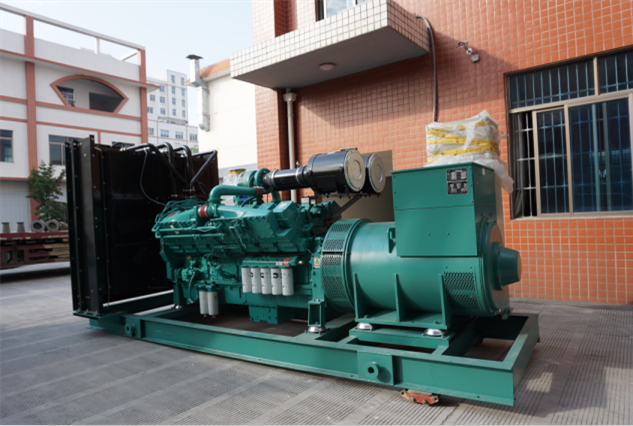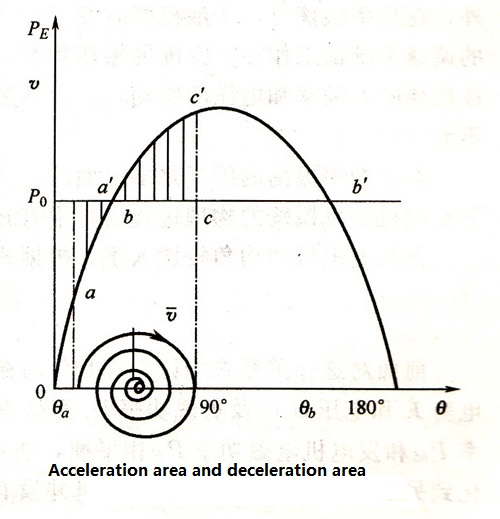# Dynamic Stability Concept of Parallel Operation of Diesel Generator Sets

Dec. 17, 2018

Static stability is only a requirement for parallel operation of the generator, and it does not indicates the ability of the system to maintain synchronous operation in the event of a sharp disturbance. Take the short circuit in the grid as an example to illustrate this problem. When the power grid is short circuited, the power angle characteristics of each generator and its output power will suddenly change. For example, a short circuit occurs near the busbar, the bus voltage is close to zero, and the generators are completely lost in contact and influence.Since the diesel governor has a certain inertia, the prime mover does not change immediately. The generator rotors are accelerated (and possibly decelerated) under the residual power of the diesel engine. The acceleration of each generator set depends on the mechanical inertia of the genset, electrical characteristics, operating parameters before short circuit and short circuit impedance and short circuit duration. Therefore, after the short circuit is cut off, not only the relative position of each generator rotor changes, but also the rotational speed is not consistent. Whether the generator rotor can resume synchronous operation after a certain relative oscillation depends on the kinetic energy of each genset. And the size and direction of the residual torque of the diesel engine. In order to qualitatively explain the problem, only the switching process of one generator rotor is relatively seen, regardless of the influence of other generator sets dynamic processes.

It is assumed that after the short circuit is cut off, a generator has the power angle characteristic shown in figure, and the power angle of the generator is θa at this time, and it is assumed that the voltage vector of the generator rotor and the grid has the same rotational speed at this moment. The speed V is zero. Then, under the action of the residual power of the diesel engine, the generator rotor is accelerated by θa, and the θ angle is gradually increased. Before reaching point b, the diesel engine is always greater than the generator torque, and the acceleration process continues until point b. when θ=θb, the generator output power and the diesel engine power are balanced, the residual torque is zero, and the relative rotational speed V is no longer increased to reach a maximum value.Since the speed of the generator rotor is greater than the speed of the voltage vector at this time, the generator rotor will continue to increase the angle θ by inertia over point b, but the residual power changes the sign, and the generator rotor begins to decelerate until point c. The relative velocity V is zero and the angle θ reaches a maximum value. Under the action of the residual torque, the rotor starts the opposite motion process. The kinetic energy obtained by the generator during the acceleration process can be expressed by the acceleration area a'ab. The kinetic energy consumed during the deceleration can be expressed by the deceleration area bcc. according to the principle of energy conservation, the acceleration area and the deceleration area should be equal. Obviously, the maximum value of the deceleration area is bcbb. If the acceleration area is smaller than this maximum value (as shown in figure), the generator rotor can be stabilized after a number of oscillations under the action of the damping factor. 6 points, indicating that the system has a stability after being disturbed by a short circuit, if the acceleration area is greater than bcb'b, the motion of the generator rotor will exceed the 6 point, and the residual torque of the diesel engine changes the sign again to make the generator. The rotor is further accelerated until it loses synchronization, so the system does not have dynamic stability.

Similar situations and problems can occur when the system is subjected to other large disturbances. For example, if the frequency difference of the input is too high or the deceleration swinging process will occur under the residual power of the diesel engine, and the deceleration area cannot be offset by the acceleration area. In the case of the parallel unit, the dynamic stability will also be lost. When the high power asynchronous motor starts, the parallel genset is suddenly disassembled, etc., the dynamic stability problem of the parallel diesel generator set is also generated.

For diesel power stations, because the capacity of the power station is small, and the power of a single generator and the power of the power station are relatively close, the bus voltage also needs to change drastically during the dynamic process. Therefore, dynamic stability analysis usually requires calculation of each generator set. The movement of the rotor of the genset and the relative motion between the rotors. In addition, most diesel power plants have asynchronous motor loads comparable to the capacity of the power plant. Since the torque of the asynchronous motor is proportional to the square of the voltage, when the bus voltage decreases, the torque drops sharply, and the asynchronous motor is rapidly decelerated or even stopped. Turning, this in turn greatly increase the power drawn by the motor from the grid, which in turn affects the stability of the generator.